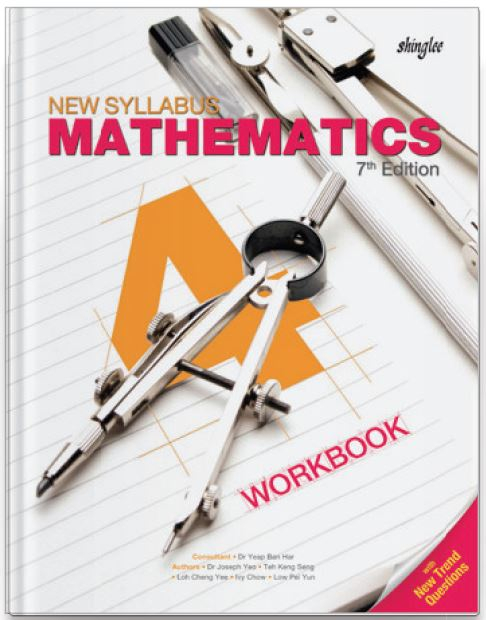Our prices are denominated in USD.

We ship internationally!# New Syllabus Mathematics Workbook 4 (7th Ed)

\$11.25

New Syllabus Mathematics is the most widely-adopted series of secondary mathematics textbooks in Singapore. Following the three-part lesson approach, pupils begin with an anchor task, followed by a guided practice, before concluding with an independent practice. New concepts are introduced and built upon in the textbooks. Well-structured exercises developed in
accordance to the lesson objectives are found in the workbooks.

This is a 4-year course in preparation for Cambridge O-level.

This is a 4-year course.

For Secondary 4 / Grade 10 / Year 10

1. Sets
Venn Diagrams, Universal Set, Complements, Subsets, Intersection and Union of Sets

2. Probability of Combined Events
Possibility and Tree Diagrams, Addition and Multiplication Laws, Mutually Exclusive Events and Independent Events

3. Statistical Data Analysis
Cumulative Frequency Table and Curve, Median, Quartiles, Percentiles, Range, Interquartile Range, Box-and-Whisker Plots and Standard Deviation

4. Matrices

5. Vectors
Addition, Subtraction and Scalar Multiples of Vectors, Position Vectors and Applications

6. Revision: Numbers and Algebra
• Numbers, Percentages, Proportion, Ratio, Rate and Speed
• Algebraic Manipulation, Formulae, Equations and Inequalities
• Functions and Graphs
• Sets
• Matrices

7. Revision: Geometry and Measurement
• Angles, Triangles, Polygons
• Congruence Similarity
• Pythagoras' Theorem, Trigonometry  and Mensuration
• Coordinate Geometry
• Vectors
• Properties of Circles

8. Revision: Probability and Statistics